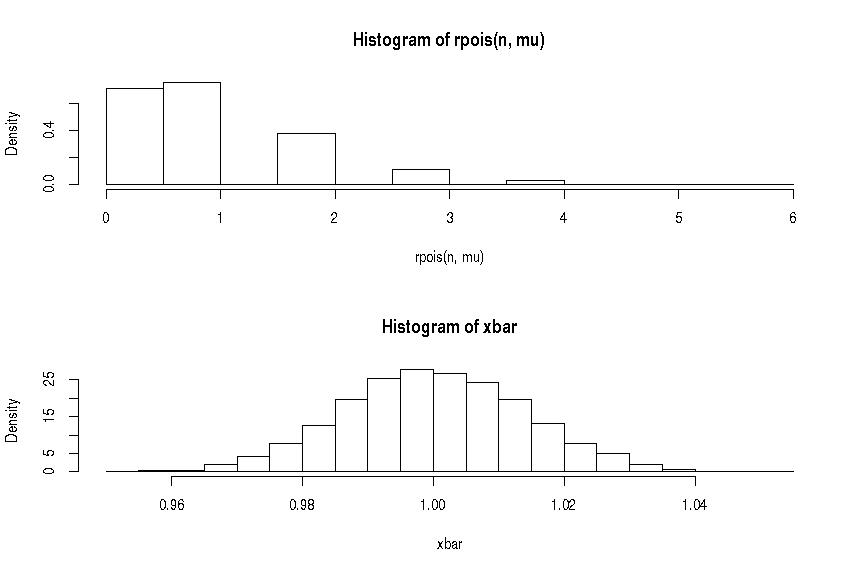## Sunday, June 15, 2014

### Lies, Damned Lies and Performance Statistics

A new way of measuring performance

I've introduced HdrHistogram to my pet project, JStringServer. The advantages of HdrHistogram is that it keeps the results in constant memory with constant access time. The only cost is some approximation of results and since I'm only interested in means, standard deviations etc I can live with that.

HdrHistogram works by keeping an array of "buckets" which represent a range of values. Any given value that falls into a particular bucket merely increases that bucket's counter. This is much more memory efficient than, say Apache's JMeter that keeps every reading in memory.

The range of these buckets grow exponentially (well, there are sub-buckets but let's keep this simple). So, all results that are outliers are collected in just one or two buckets. This is very memory efficient as each bucket is a Java long each.

Means, standard deviations and all that

Most performance tools like Apache's JMeter will give you a mean and a standard deviation. You might have even heard that 68% of results are within a standard deviation of the mean, 95% within 2 standard deviations, 99.7% within 3 etc (see here for the rule). But this is only true for a normal distribution (the typical, bell-shaped curve).

The time it takes for a given measurement independent of all others will typically conform to a Poisson distribution. (See Mathematical Methods in the Physical Sciences by Mary Boas for a clear derivation of this formula from first principles.)

Results

Typically, I see:

Initiated 412759 calls. Calls per second = 10275. number of errors at client side = 0. Average call time = 10ms
ThreadLocalStopWatch [name=read, totalCallsServiced=412659, max time = 630ms, Mean = 6.125236575477573, Min = 0, standard deviation 8.263969133274433, Histogram with stepsize = 1. Approximate values: 791, 1822, 3524, 15650, 143582, 307867, 243841, 81151, 18447, 4716, 1395, 493, 314, 195, 106, 97, 82, 66, 35, 10, 6, 7, 10, 7, 12, 14, 8, 7, 4, 2]
ThreadLocalStopWatch [name=write, totalCallsServiced=412719, max time = 26ms, Mean = 0.08618696982692825, Min = 0, standard deviation 0.327317028847167, Histogram with stepsize = 1. Approximate values: 411831, 33604, 771, 238, 80, 32, 17, 8, 1, 1, 2, 4, 5, 3, 0, 0, 1, 1, 1, 1, 0, 0, 1, 2, 5, 8, 4, 0, 0, 0]
ThreadLocalStopWatch [name=connect, totalCallsServiced=412720, max time = 3005ms, Mean = 4.17902209730568, Min = 0, standard deviation 65.54450133531354, Histogram with stepsize = 1. Approximate values: 408721, 117052, 2056, 852, 282, 114, 57, 30, 15, 12, 10, 10, 15, 10, 3, 5, 4, 3, 2, 1, 3, 4, 2, 0, 1, 1, 0, 1, 1, 0]
ThreadLocalStopWatch [name=total, totalCallsServiced=410682, max time = 66808064ns, Mean = 6295825.973268021, Min = 854104, standard deviation 1089344.7561186429, Histogram with stepsize = 1333333. Approximate values: 145, 837, 1596, 25512, 273990, 93021, 12099, 2283, 520, 273, 126, 77, 80, 55, 11, 9, 5, 9, 10, 11, 15, 5, 3, 2, 3, 3, 0, 0, 4, 1, 2]

For something that approximates to a Poisson distribution, we'd expect the mean and standard deviation to be about the same. Since this is not true for the total time, perhaps this is not a Poisson distribution. The results for reading data does have these two values roughly the same so let's look at them.

Let's see if the distribution of the values conform to the Poisson distribution or even the Normal (aka Gaussian). In the R language:

require(graphics)

# Histogram of times. Each interval is 1ms starting from 1ms
xread <- c(791, 1822, 3524, 15650, 143582, 307867, 243841, 81151, 18447, 4716, 1395, 493, 314, 195, 106, 97, 82, 66, 35, 10, 6, 7, 10, 7, 12, 14, 8, 7, 4, 2)

par(mfrow = c(3, 1)) # we're looking at 3 graphs

ac <- barplot(x, main="Actual Distribution", axes=TRUE)
axis(1, at=ac, labels=TRUE)

<- 1:length(x)

expectedPoisson = n * dpois(i, lambda=mean)
expectedNorm    = n * dnorm(i, mean=mean, sd=sd)

nm <- barplot(expectedNorm, main="Expected Normal Distribution")
axis(1, at=nm, labels=TRUE)

pn <- barplot(expectedPoisson, main="Expected Poisson Distribution")
axis(1, at=pn, labels=TRUE)

chisq.test(x, p=expectedPoisson, rescale.p=TRUE)
chisq.test(x, p=expectedNorm,    rescale.p=TRUE)

The last two lines are testing whether the actual distribution conforms to the Poisson (give a lambda value) or a Normal (given the mean and the standard deviation). It does this using the chi-squared test (basically, a comparison of the squared differences between what we expect and what we get).

For these particular results, the p-value from the chi-squared tests tell me they neither conform to a Poisson not a Normal distribution (in fact, they could barely be further from them). Looking at the graphs, you can see this by-eye:
So, what went wrong? Well, the Poisson distribution relies on events being independent of each other which is clearly not the case when there is contention for resources.

The Central Limit Theorem

(Aside):

The means of a distribution form a normal distribution given enough runs even if that original distribution is not itself a normal distribution.

Let's look at plotting the Poisson distribution in R:

mu=1; sigma=10   # mean, standard deviation
n=5000           # Number of iterations
xbar=rep(0,n)    # Holds the results of the iterations

for (i in 1:n) {

xbar[i]=mean(
rpois(5000, mu) # num. random variables = 5000, mu = means
)
}

par(mfrow = c(2, 1))

# Plot a typical Poisson distribution

hist(rpois(n, mu),prob=TRUE,breaks=15)

# Plot the results of n Poisson distributions

hist(xbar,prob=TRUE) #,xlim=c(70,130),ylim=c(0,0.1))

Gives this result:A typical Poisson distribution and the mean of a Poisson over many iterations
This is quite nice as it means that if you run performance tests repeatedly, your means will approach a Normal Distribution giving some predictive power over them.